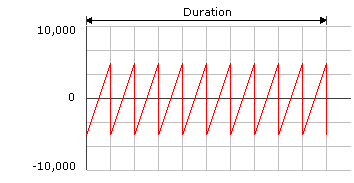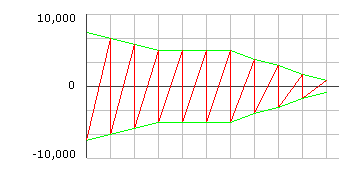# Basic Concepts of Force Feedback

A particular instance of force feedback is called an effect, and the push or resistance is called the force. Most effects fall into one of the following categories:

• Constant force. A steady force in a single direction.

• Ramp force. A force that steadily increases or decreases in magnitude.

• Periodic effect. A force that pulsates according to a defined wave pattern.

• Condition. A reaction to motion or position along an axis. Two examples are a friction effect that generates resistance to movement of the joystick, and a spring effect that pushes the stick back toward a certain position after it has been moved from that position.

The strength of the force is called its magnitude. Magnitude is measured in units ranging from 0 (no force) through 10,000 (maximum force for the device, defined for C/C++ and Microsoft Visual Basic as DI_FFNOMINALMAX). A negative value indicates force in the opposite direction. Magnitudes are linear: a force of 10,000 is twice as great as one of 5,000.

Ramp forces have a beginning and ending magnitude. For a periodic effect, the basic magnitude is the force at the peak of the wave.

The direction of a force is the direction from which it comes. A positive force on a given axis pushes from the positive toward the negative.

Effects also have duration, measured in microseconds. Periodic effects have a period, or the duration of one cycle, also measured in microseconds. The phase of a periodic effect is the point along the wave at which playback begins.

The following illustration represents a sawtooth periodic effect with a magnitude of 5,000, or half the maximum force for the device. The horizontal axis represents the duration of the effect, and the vertical axis represents the magnitude. Points above the center line represent positive force in the direction defined for the effect, and points below the center line represent negative force, or force in the opposite direction.A force may be further shaped by an envelope. An envelope defines an attack value and a fade value, which modify the beginning and ending magnitude of the effect. Attack and fade also have duration, which determines how long the magnitude takes to reach or fall away from the sustain value, the magnitude in the middle portion of the effect.

The following diagram shows an envelope. The attack level is set to 8,000 and the fade level to 1,000. The sustain level is defined by the basic magnitude of the force to which the envelope is being applied; in the example, it is 5,000. In this case, the attack is greater than the sustain, giving the effect an initial strong kick. Both the attack and the fade level can be either greater or less than the sustain level.The next diagram shows the result of the envelope being applied to the periodic effect in the first diagram. The envelope is mirrored on the negative side of the magnitude. An attack value of 8,000 means that the initial magnitude of the force in either direction is 80 percent of the maximum possible.Periodic effects and conditions can also be modified by the addition of an offset, which defines the amount by which the waveform is shifted up or down from the base level. The practical effect of applying a positive offset to the sawtooth example would be to strengthen the positive force and weaken the negative one - in other words, the force would peak more strongly in one direction than in the other.

Finally, the overall magnitude of an effect can be scaled by gain, which is analogous to a volume control in audio. A single gain value can be applied to all effects for a device. You might want to do this to compensate for stronger or weaker forces on different hardware or to accommodate the user's preferences.